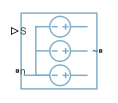# Controlled Voltage Source (Three-Phase)

Ideal three-phase controlled voltage source

Since R2019a

•Libraries:
Simscape / Electrical / Sources

## Description

The Controlled Voltage Source (Three-Phase) block represents an ideal three-phase voltage source that maintains the specified voltage regardless of the current through it.

To choose the input type of this block, set the Input type parameter to `Instantaneous` or ```Sinusoidal magnitude and phase shift```. If you select `Instantaneous`, this block works only in time simulation mode. If you select `Sinusoidal magnitude and phase shift`, this block works in both time and frequency-time simulation modes. For more information, see Frequency and Time Simulation Mode.

To access the implementation with an expanded, three-phase port, double-click the block and set the Modeling option parameter to `Expanded three-phase ports`.

## Ports

### Input

expand all

Physical signal input port associated with the control signal. The output voltages are [va vb vc] = S. For an ideal sinusoidal source, input three sine waves that have the requisite amplitude and phase delays of $\left[\begin{array}{ccc}0& -\frac{2\pi }{3}& \frac{2\pi }{3}\end{array}\right]$.

#### Dependencies

To enable this port, set Input type to `Instantaneous`.

Physical signal input port associated with the phase-to-phase RMS voltage.

#### Dependencies

To enable this port, set Input type to ```Sinusoidal magnitude and phase shift```.

Physical signal input port associated with the phase shift, in radians.

#### Dependencies

To enable this port, set Input type to ```Sinusoidal magnitude and phase shift```.

### Conserving

expand all

Electrical conserving port associated with the neutral phase.

Expandable three-phase port associated with the output voltage.

#### Dependencies

To enable this port, set Modeling option to `Composite three-phase ports`.

Electrical conserving port associated with the a-phase output voltage.

#### Dependencies

To enable this port, set Modeling option to `Expanded three-phase ports`.

Electrical conserving port associated with the b-phase output voltage.

#### Dependencies

To enable this port, set Modeling option to `Expanded three-phase ports`.

Electrical conserving port associated with the c-phase output voltage.

#### Dependencies

To enable this port, set Modeling option to `Expanded three-phase ports`.

## Parameters

expand all

Whether to model composite or expanded three-phase ports.

Composite three-phase ports represent three individual electrical conserving ports with a single block port. You can use composite three-phase ports to build models that correspond to single-line diagrams of three-phase electrical systems.

Expanded three-phase ports represent the individual phases of a three-phase system using three separate electrical conserving ports.

Type of physical signal input. Choose between:

• `Instantaneous` — The output voltages, [va vb vc], are equal to the values of the input port S.

• ```Sinusoidal magnitude and phase shift``` — The output voltages are equal to:

`$\begin{array}{l}{v}_{a}=\left(\sqrt{2}\frac{Vt}{\sqrt{3}}\right)\mathrm{sin}\left(2\pi {F}_{rated}+ph\right)\\ {v}_{b}=\left(\sqrt{2}\frac{Vt}{\sqrt{3}}\right)\mathrm{sin}\left(2\pi {F}_{rated}+ph-2\frac{\pi }{3}\right)\\ {v}_{c}=\left(\sqrt{2}\frac{Vt}{\sqrt{3}}\right)\mathrm{sin}\left(2\pi {F}_{rated}+ph+2\frac{\pi }{3}\right)\end{array}$`

Rated electrical frequency. The value of this parameter must be equal to the value of the rated electrical frequencies of other sinusoidal sources in your model, if present.

#### Dependencies

To enable this parameter, set Input type to ```Sinusoidal magnitude and phase shift```.

## Version History

Introduced in R2019a